## Online Registration is open until 8 pm on 5/25!## This new course includes ALL the trails at Rope Mill Park!

Make plans to join us on May 27th for our newest event! This Fast+Furious 15 course features all the trails at Rope Mill Park and will begin along the concrete path to allow riders to space out before entering the singletrack portion of the course.

Riders will turn right at the bridge and complete Moore's Pass, Power House, Raceway and Turbine all in a clockwise direction. These trails are farily wide and allow for passing.

Riders will then return across the bridge and complete Explorer Loops 1, 2, and 3 counter-clockwise. The course returns via the singletrack trail that links Explorer to the parking lot, crosses the parking lot, then heads onto the Avalanche Trail where riders will proceed counter-clockwise on Avalance Trail Loops 1, 2, and 3 and then head back to the start/finish to complete the course.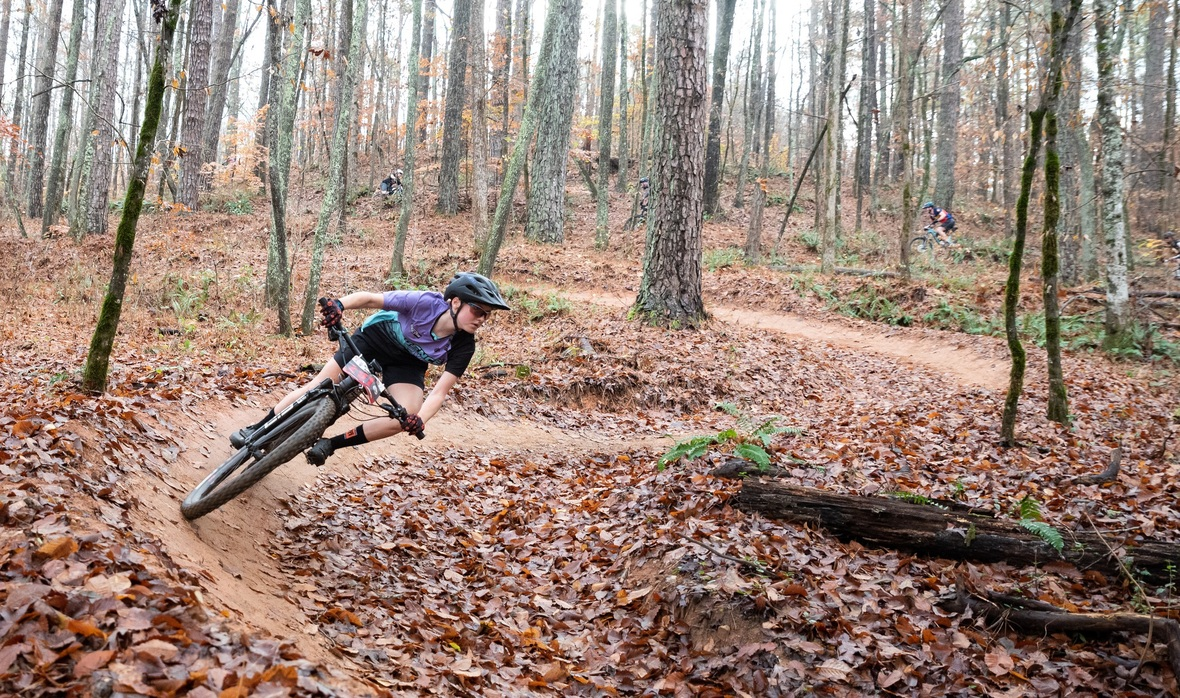You can never have too many berms!!

The categories for 2023 are age based and the same as we have used for the Blankets Creek Dirty 15 Mile Race. You can see full details and a list of categories on the ROPE MILL FAST+FURIOUS 15 WEBSITE.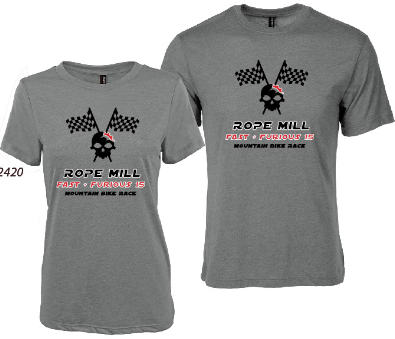2023 Race Shirt Design (grey tri-blend shirts)

# We have the SWAG - Register by May 5th Shirt Size Deadline!

## Mountain Goat Race Gear Tote and FREE race photo downloads courtesy of our swag sponsor, Dalton Automotive!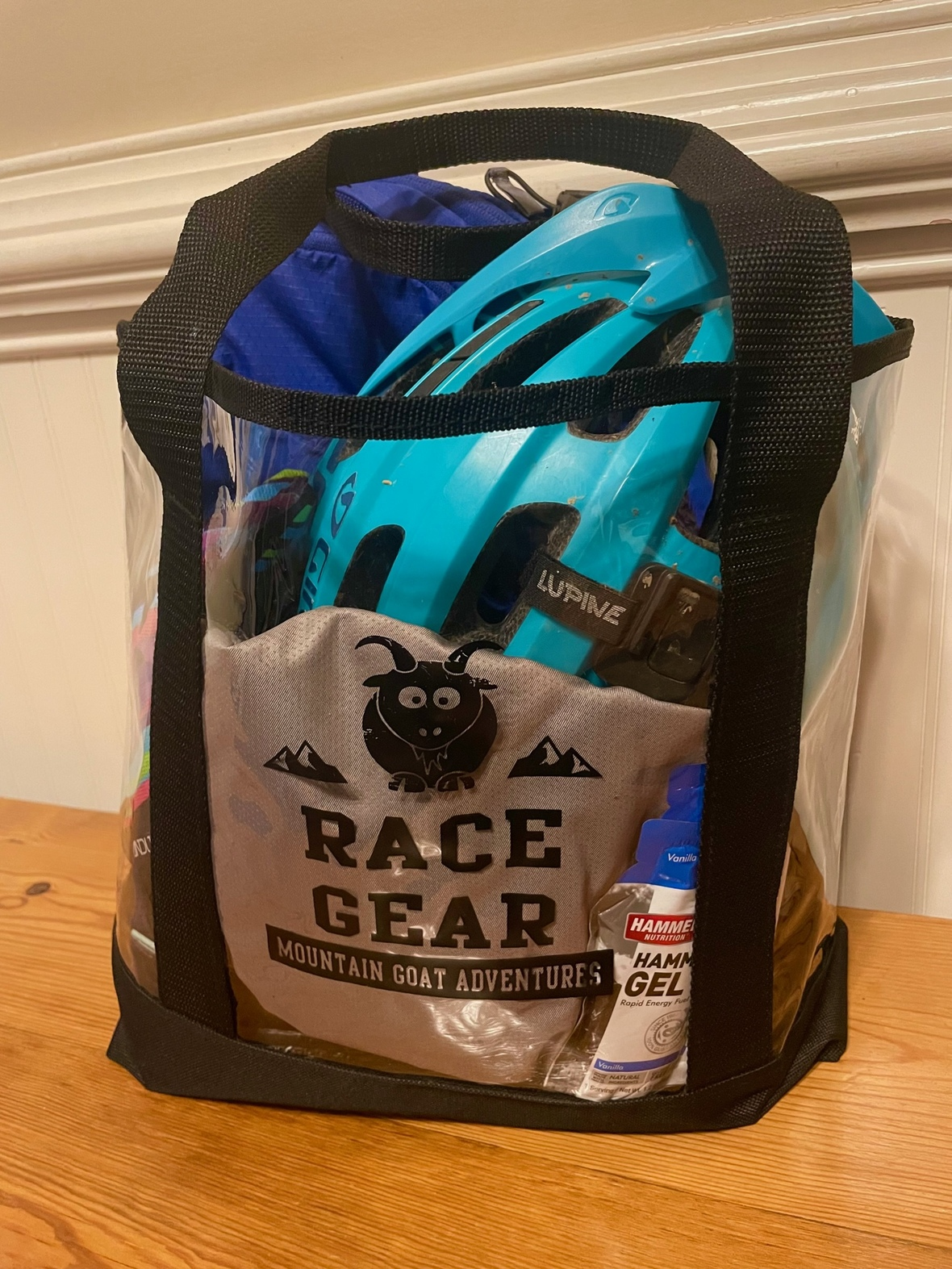Clear Plastic Gear Totes!
▪ Medals and sponsor prizes 3 deep in all categories
▪ Podium shirts for all first place finishers.
▪ Staggered starts by category for rider/volunteer safety.
▪ Post race snacks and fluids
 ▪ Medals and sponsor prizes 3 deep in all categories
 ▪ Podium shirts for all first place finishers.
 ▪ Staggered starts by category for rider/volunteer safety.
 ▪ Post race snacks and fluids

## HAVE FAMILY MEMBERS WHO RUN?

We are also offering a separate 10k or half marathon trail run on a similar course in the morning before the bike race!

This event is a major fundraiser for SORBA Woodstock, so please come out and support your local trails!

 table div table+table+table+table+table+table+table+table div table{width:100%;padding:0}table div table+table+table+table+table+table+table+table div table img{width:96.23%;padding:0;float:none}table div table+table+table+table+table+table+table+table div table td{width:100%;padding:0 1.88% 18px}/* styles */table div table+table+table+table+table+table+table+table+table div table td,table.module-8{width:100%;padding:0}table div table+table+table+table+table+table+table+table+table div table{width:100%;float:none;margin-left:auto;margin-right:auto;padding:0}table div table+table+table+table+table+table+table+table+table div table a{border:0 none;text-decoration:none}table div table+table+table+table+table+table+table+table+table div table img{width:100%!important;border:0 none;text-decoration:none}/* styles */

## Blankets Creek 3 and 6 Hour Mountain Bike Race presented by Free-Flite Bicycles - September 30, 2023 at Blankets Creek Trails in Canton, GA!

 table div table+table+table+table+table+table+table+table+table+table+table div table,table.module-10{width:31.13%;float:left;padding:0}table div table+table+table+table+table+table+table+table+table+table+table div table a{border:0 none;text-decoration:none}table div table+table+table+table+table+table+table+table+table+table+table div table img{width:100%!important;border:0 none;text-decoration:none}table div table+table+table+table+table+table+table+table+table+table+table div table td{width:100%;padding:0 20px 20px 0}/* styles */ Make plans to join us for the Blankets Creek 3 and 6 Hour Race presented by Free-Flite Bicycles on September 30th! Solo, 2 and 3 person team categories are available in the 6 Hour Race.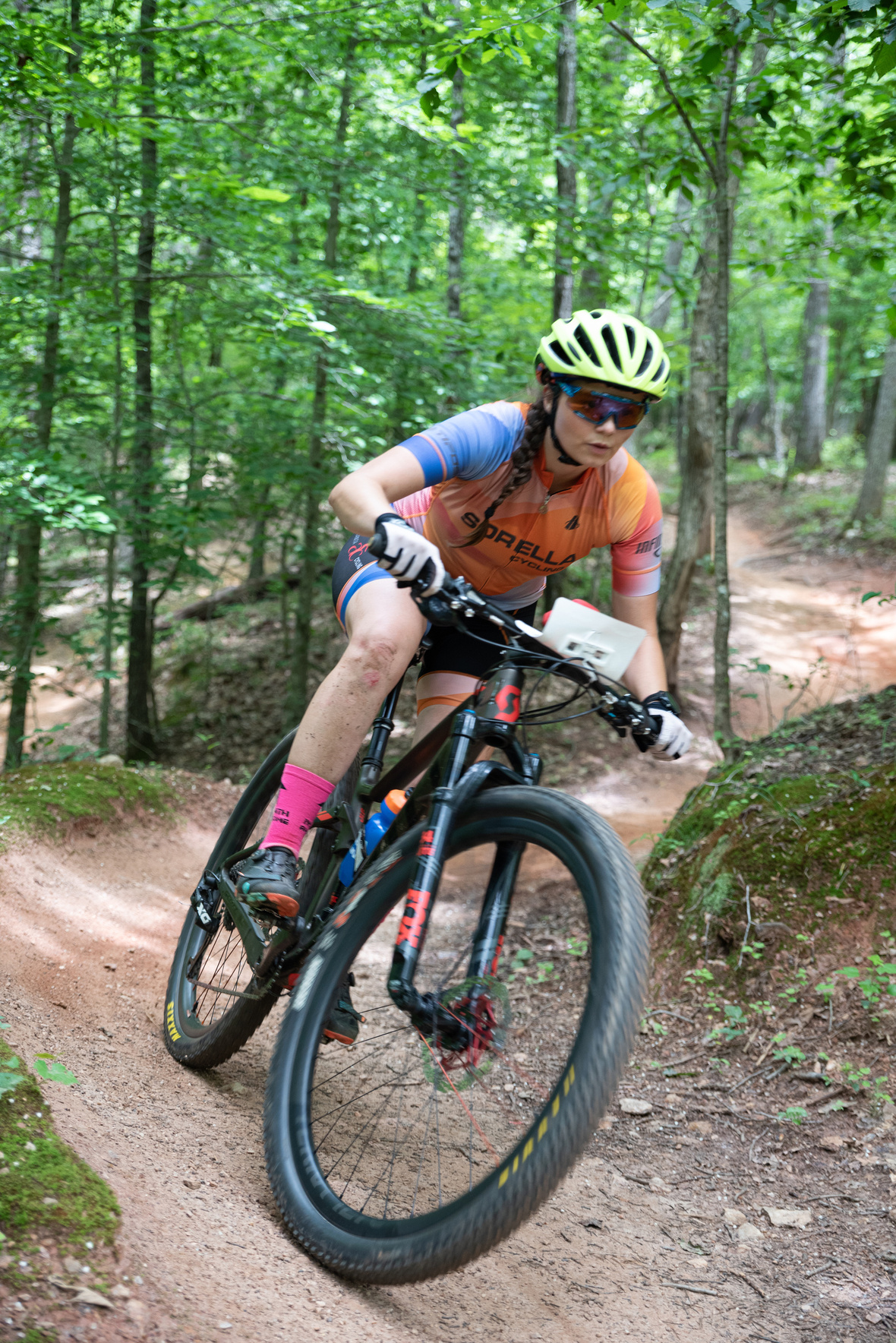The 3 Hour Option has a TON of categories for those who wish to ride 3 hours solo, as well as a one and two lap option for junior riders who aren't ready for a full 3 hour event. You can check out the available Categories and FULL EVENT DETAILS HERE

Medals and sponsor prizes 3 deep in all categories, podium shirts for all first place finishers.

The 2023 race course will be ridden CLOCKWISE on both Dwelling and VMT.
COURSE DETAILS AND MAP HERE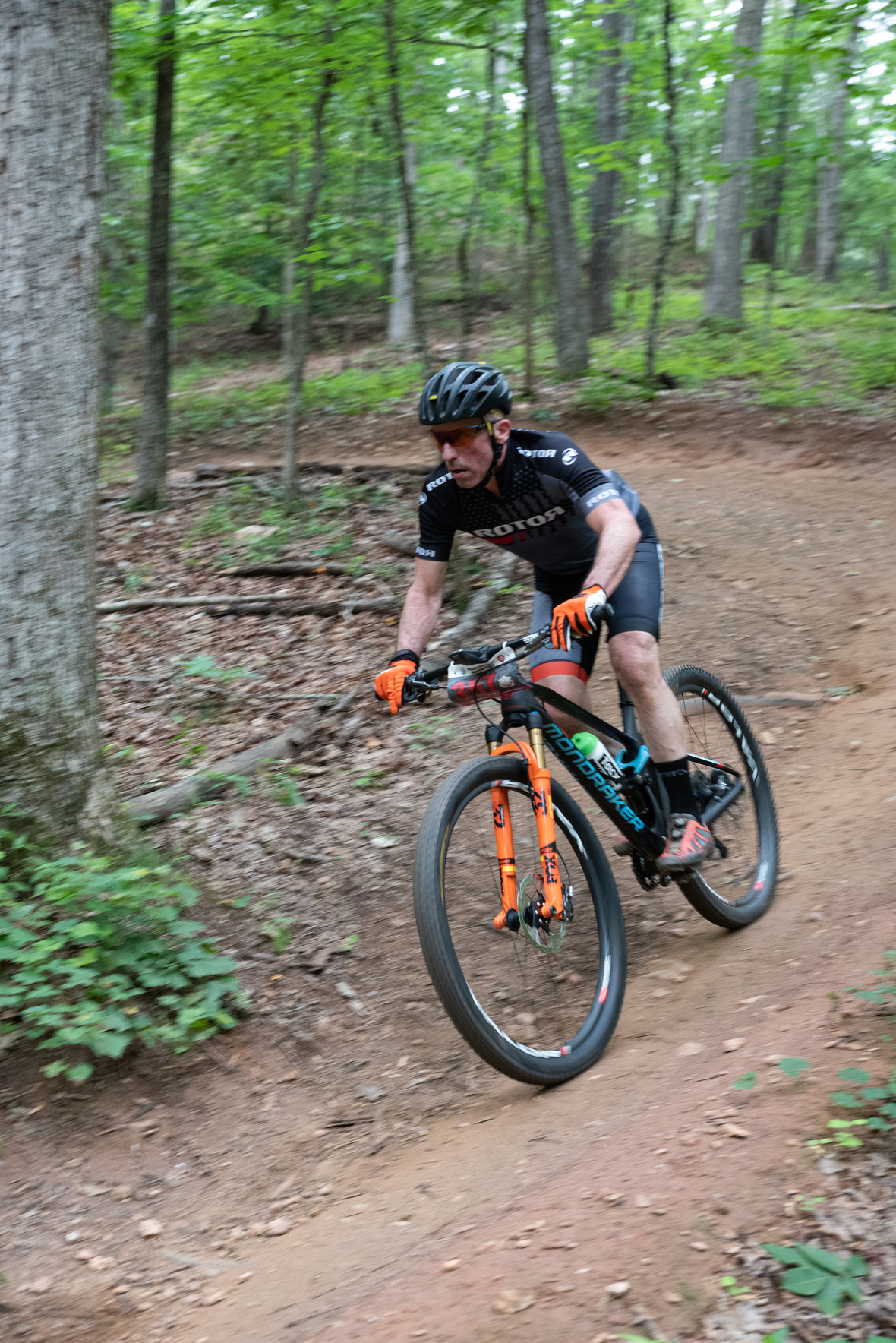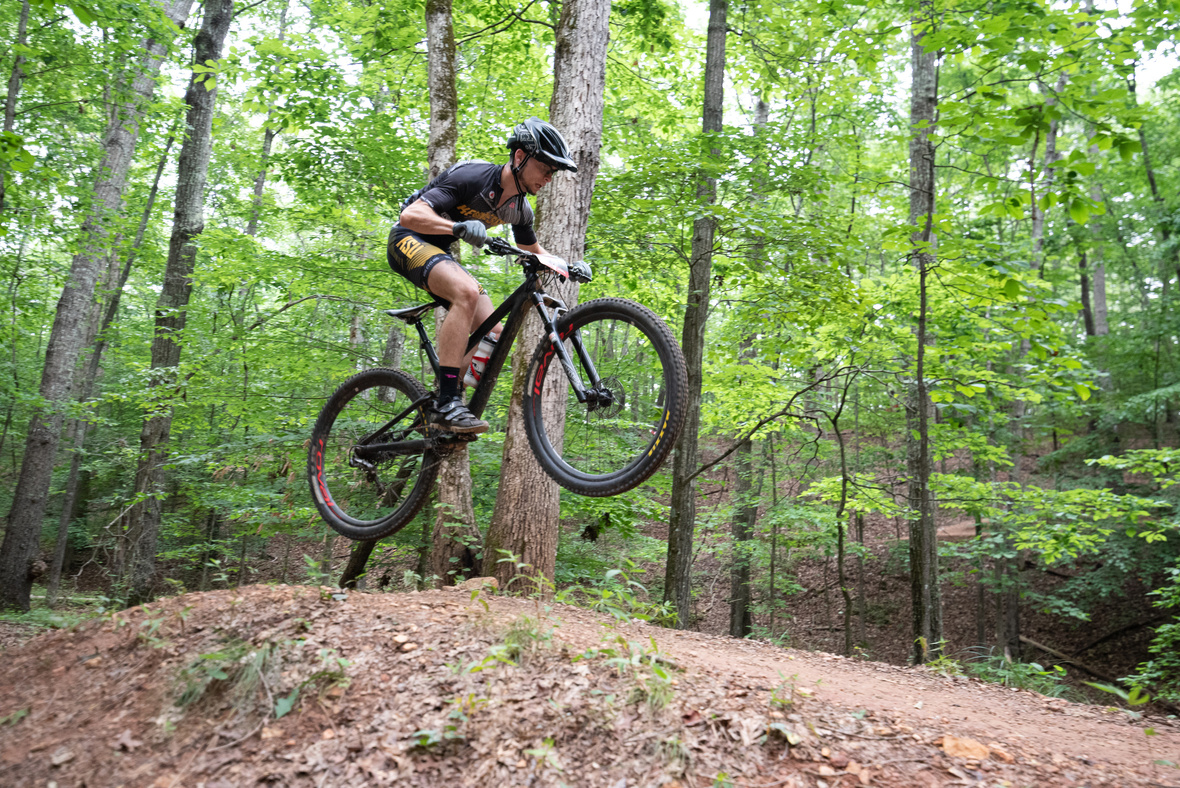table div table+table+table+table+table+table+table+table+table+table+table+table+table+table+table div table{width:100%;padding:0}table div table+table+table+table+table+table+table+table+table+table+table+table+table+table+table div table img{width:96.23%;padding:0;float:none}table div table+table+table+table+table+table+table+table+table+table+table+table+table+table+table div table td{width:100%;padding:0 1.88% 18px}/* styles */table div table+table+table+table+table+table+table+table+table+table+table+table+table+table+table+table div table td,table.module-15{width:100%;padding:0}table div table+table+table+table+table+table+table+table+table+table+table+table+table+table+table+table div table{width:100%;float:none;margin-left:auto;margin-right:auto;padding:0}table div table+table+table+table+table+table+table+table+table+table+table+table+table+table+table+table div table a{border:0 none;text-decoration:none}table div table+table+table+table+table+table+table+table+table+table+table+table+table+table+table+table div table img{width:100%!important;border:0 none;text-decoration:none}/* styles */

## Rope Mill Dirty Duathlon and Trail Run presented by Northside Hospital - Cherokee - November 11, 2023 at Rope Mill Park in Woodstock, GA!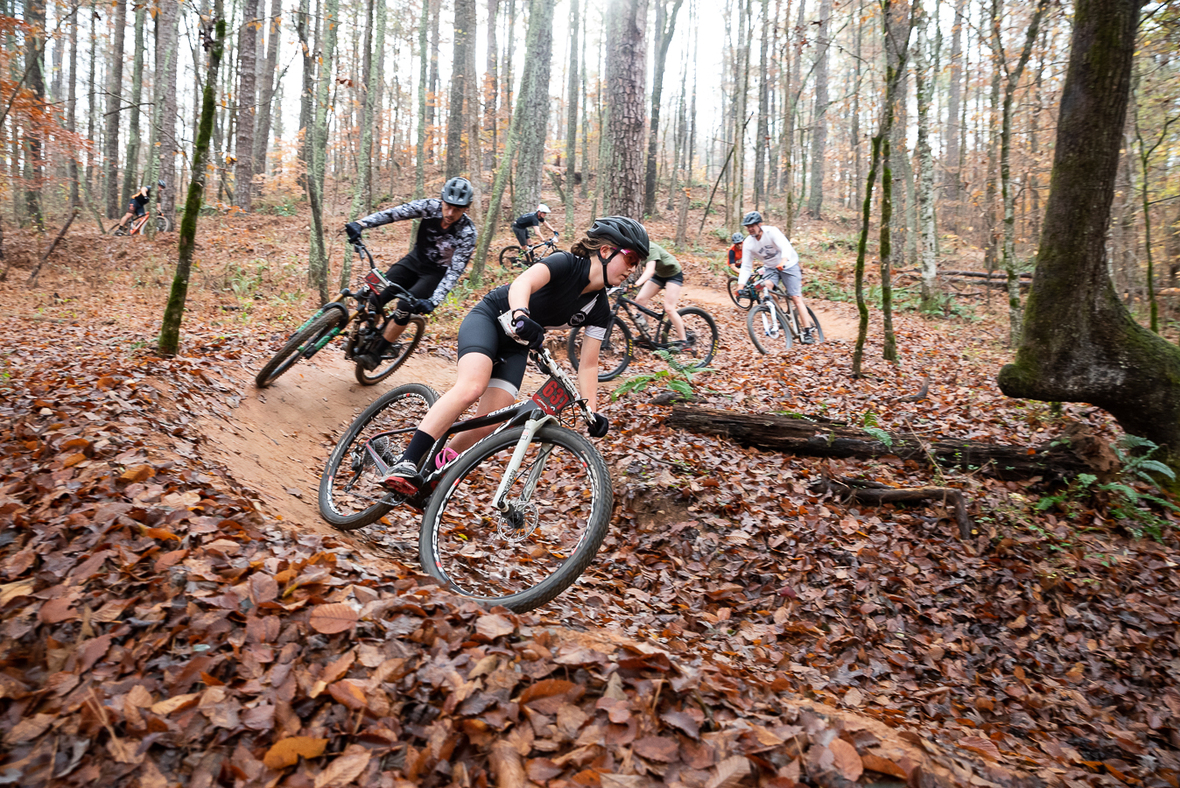# This is everyone's favorite event where you can race as a solo or on a team. Pair up with your spouse or kids!

The opening bike loop will be 5.5 miles on the Mill Trails, followed by a 3.3 mile trail run on Explorer, and finally a 5.5 mile bike on the Avalanche Trails. The course covers all the trails at Rope Mill Park!

Solo, 2 and 3 person beginner/family team categories are available in the duathlon. We will be using traditional mountain bike race categories, as we have done in the past, to help alleviate congestion on the opening bike loop. The run is short enough that those who do not typically run still have plenty of time to start training!

All participants receive a super comfy t-shirt and those that register by October 20th also receive a commemorative swag item. Prizes and awards presented to the top 3 in each duathlon category. Post race snacks and drinks available.

## HAVE FAMILY MEMBERS WHO ONLY WANT TO RUN?

We are also offering a separate 3.3 mile trail run on the Explorer Trails at this event for those who would prefer to just participate in a run.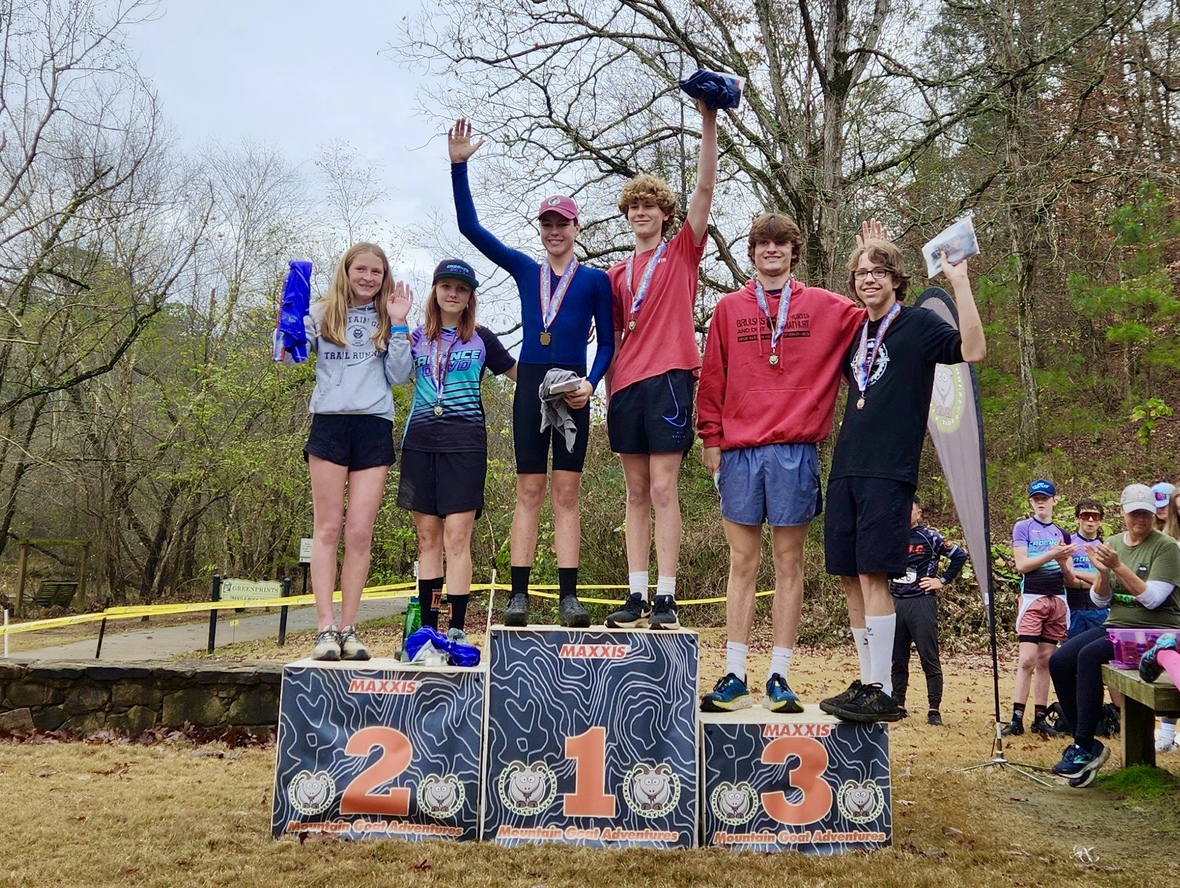table div table+table+table+table+table+table+table+table+table+table+table+table+table+table+table+table+table+table+table+table+table div table{width:100%;padding:0}table div table+table+table+table+table+table+table+table+table+table+table+table+table+table+table+table+table+table+table+table+table div table img{width:96.23%;padding:0;float:none}table div table+table+table+table+table+table+table+table+table+table+table+table+table+table+table+table+table+table+table+table+table div table td{width:100%;padding:0 1.88% 18px}/* styles */## Volunteer Opportunities and Trail Work Parties!

We are partnering with SORBA Woodstock to bring you these events. SORBA Woodstock has built and maintains the trails at both Blankets Creek and Rope Mill Park. These events serve as major fundraisersfor the Chapter - please come out and show your support by either racing or volunteering!

## Want to volunteer at these events for SORBA Woodstock? We need you!

Please send an email directly to lisa@mountaingoatadventures.com with your shirt size and availability. Volunteers are needed for registration, parking, course marshaling and bike patrol.

## Want to help work on your local trails?

Visit the SORBA Woodstock Website and view the calendar for work party dates and locations. Wear long pants and closed-toe shoes, and bring your own water. SORBA Woodstock provides the rest. This is a chance to help give back to the trails. SORBA Woodstock could not maintain our wonderful trails without the help of a small army of generous volunteers like you!

 table div table+table+table+table+table+table+table+table+table+table+table+table+table+table+table+table+table+table+table+table+table+table+table+table div table{width:100%;padding:0}table div table+table+table+table+table+table+table+table+table+table+table+table+table+table+table+table+table+table+table+table+table+table+table+table div table img{width:96.23%;padding:0;float:none}table div table+table+table+table+table+table+table+table+table+table+table+table+table+table+table+table+table+table+table+table+table+table+table+table div table td{width:100%;padding:0 1.88% 18px}/* styles */# We're excited to announce Hammer Nutrition as a sponsor for this year's SORBA Woodstock events!

Hammer has been supplying athletes with natural products free of added sugars, artificial colors, flavors, chemicals, and preservatives for over 35 years. Visit their site to learn how to fuel smart, so come race day, you’re ready to perform at your best!

If you'd like to train with the product that will be on course, Hammer's USA-based nutrition reps are happy to answer your questions and find the perfect product for your needs.

Take a look at how Hammer Nutrition provides you energy, endurance, and recovery with their fuels, supplements, and recovery products here.

 table div table+table+table+table+table+table+table+table+table+table+table+table+table+table+table+table+table+table+table+table+table+table+table+table+table+table div table{width:100%;padding:0}table div table+table+table+table+table+table+table+table+table+table+table+table+table+table+table+table+table+table+table+table+table+table+table+table+table+table div table img{width:96.23%;padding:0;float:none}table div table+table+table+table+table+table+table+table+table+table+table+table+table+table+table+table+table+table+table+table+table+table+table+table+table+table div table td{width:100%;padding:0 1.88% 18px}/* styles */## Check out Mulberry Gap Adventure Basecamp for a Weekend in the North Georgia Mountains!

 table div table+table+table+table+table+table+table+table+table+table+table+table+table+table+table+table+table+table+table+table+table+table+table+table+table+table+table+table div table td,table.module-27{width:100%;padding:0}table div table+table+table+table+table+table+table+table+table+table+table+table+table+table+table+table+table+table+table+table+table+table+table+table+table+table+table+table div table{width:100%;float:none;margin-left:auto;margin-right:auto;padding:0}table div table+table+table+table+table+table+table+table+table+table+table+table+table+table+table+table+table+table+table+table+table+table+table+table+table+table+table+table div table a{border:0 none;text-decoration:none}table div table+table+table+table+table+table+table+table+table+table+table+table+table+table+table+table+table+table+table+table+table+table+table+table+table+table+table+table div table img{width:100%!important;border:0 none;text-decoration:none}/* styles */
 table div table+table+table+table+table+table+table+table+table+table+table+table+table+table+table+table+table+table+table+table+table+table+table+table+table+table+table+table+table div table td,table.module-28{width:100%;padding:0}table div table+table+table+table+table+table+table+table+table+table+table+table+table+table+table+table+table+table+table+table+table+table+table+table+table+table+table+table+table div table{width:100%;float:none;margin-left:auto;margin-right:auto;padding:0}table div table+table+table+table+table+table+table+table+table+table+table+table+table+table+table+table+table+table+table+table+table+table+table+table+table+table+table+table+table div table a{border:0 none;text-decoration:none}table div table+table+table+table+table+table+table+table+table+table+table+table+table+table+table+table+table+table+table+table+table+table+table+table+table+table+table+table+table div table img{width:100%!important;border:0 none;text-decoration:none}/* styles */
 table div table+table+table+table+table+table+table+table+table+table+table+table+table+table+table+table+table+table+table+table+table+table+table+table+table+table+table+table+table+table div table{width:100%;padding:0}table div table+table+table+table+table+table+table+table+table+table+table+table+table+table+table+table+table+table+table+table+table+table+table+table+table+table+table+table+table+table div table img{width:96.23%;padding:0;float:none}table div table+table+table+table+table+table+table+table+table+table+table+table+table+table+table+table+table+table+table+table+table+table+table+table+table+table+table+table+table+table div table td{width:100%;padding:0 1.88% 18px}/* styles */## 2023 Full Event Calendar

 /* styles */ Want to check out our other events? Our 2023 calendar is below - we focus on mountain bike races, trail runs, and off-road duathlons. May 27, 2023 - Rope Mill 10k and 13.1 mile Trail Run May 27, 2023 - Rope Mill Fast 15 MTB Race September 9, 2023 - Fools Gold 38 and 60 Mile MTB Races September 30, 2023 - Blankets Creek 3/6 Hour Race November 11, 2023 - Rope Mill Dirty Duathlon and 5k Trail Run
 table div table+table+table+table+table+table+table+table+table+table+table+table+table+table+table+table+table+table+table+table+table+table+table+table+table+table+table+table+table+table+table+table+table div table{width:100%;padding:0}table div table+table+table+table+table+table+table+table+table+table+table+table+table+table+table+table+table+table+table+table+table+table+table+table+table+table+table+table+table+table+table+table+table div table img{width:96.23%;padding:0;float:none}table div table+table+table+table+table+table+table+table+table+table+table+table+table+table+table+table+table+table+table+table+table+table+table+table+table+table+table+table+table+table+table+table+table div table td{width:100%;padding:0 1.88% 18px}/* styles */## Special Thanks to our Sponsors!

 table div table+table+table+table+table+table+table+table+table+table+table+table+table+table+table+table+table+table+table+table+table+table+table+table+table+table+table+table+table+table+table+table+table+table+table div table{width:100%;padding:0}table div table+table+table+table+table+table+table+table+table+table+table+table+table+table+table+table+table+table+table+table+table+table+table+table+table+table+table+table+table+table+table+table+table+table+table div table table{padding:0;float:left!important;width:56.415%!important}table div table+table+table+table+table+table+table+table+table+table+table+table+table+table+table+table+table+table+table+table+table+table+table+table+table+table+table+table+table+table+table+table+table+table+table div table table+table td,table div table+table+table+table+table+table+table+table+table+table+table+table+table+table+table+table+table+table+table+table+table+table+table+table+table+table+table+table+table+table+table+table+table+table+table div table td{padding-left:0;padding-right:0}table div table+table+table+table+table+table+table+table+table+table+table+table+table+table+table+table+table+table+table+table+table+table+table+table+table+table+table+table+table+table+table+table+table+table+table div table table td{padding-left:0;padding-right:20px}table div table+table+table+table+table+table+table+table+table+table+table+table+table+table+table+table+table+table+table+table+table+table+table+table+table+table+table+table+table+table+table+table+table+table+table div table table+table{float:left!important;width:43.585%!important}/* styles */
 table div table+table+table+table+table+table+table+table+table+table+table+table+table+table+table+table+table+table+table+table+table+table+table+table+table+table+table+table+table+table+table+table+table+table+table+table div table{width:100%;padding:0}table div table+table+table+table+table+table+table+table+table+table+table+table+table+table+table+table+table+table+table+table+table+table+table+table+table+table+table+table+table+table+table+table+table+table+table+table div table table{padding:0;float:left!important;width:30.566%!important}table div table+table+table+table+table+table+table+table+table+table+table+table+table+table+table+table+table+table+table+table+table+table+table+table+table+table+table+table+table+table+table+table+table+table+table+table div table table+table td,table div table+table+table+table+table+table+table+table+table+table+table+table+table+table+table+table+table+table+table+table+table+table+table+table+table+table+table+table+table+table+table+table+table+table+table+table div table td{padding-left:0;padding-right:0}table div table+table+table+table+table+table+table+table+table+table+table+table+table+table+table+table+table+table+table+table+table+table+table+table+table+table+table+table+table+table+table+table+table+table+table+table div table table td{padding-left:0;padding-right:20px}table div table+table+table+table+table+table+table+table+table+table+table+table+table+table+table+table+table+table+table+table+table+table+table+table+table+table+table+table+table+table+table+table+table+table+table+table div table table+table{float:left!important;width:69.434%!important}/* styles */
 table div table+table+table+table+table+table+table+table+table+table+table+table+table+table+table+table+table+table+table+table+table+table+table+table+table+table+table+table+table+table+table+table+table+table+table+table+table div table{width:100%;padding:0}table div table+table+table+table+table+table+table+table+table+table+table+table+table+table+table+table+table+table+table+table+table+table+table+table+table+table+table+table+table+table+table+table+table+table+table+table+table div table table{padding:0;float:left!important;width:48.301%!important}table div table+table+table+table+table+table+table+table+table+table+table+table+table+table+table+table+table+table+table+table+table+table+table+table+table+table+table+table+table+table+table+table+table+table+table+table+table div table table+table td,table div table+table+table+table+table+table+table+table+table+table+table+table+table+table+table+table+table+table+table+table+table+table+table+table+table+table+table+table+table+table+table+table+table+table+table+table+table div table td{padding-left:0;padding-right:0}table div table+table+table+table+table+table+table+table+table+table+table+table+table+table+table+table+table+table+table+table+table+table+table+table+table+table+table+table+table+table+table+table+table+table+table+table+table div table table td{padding-left:0;padding-right:20px}table div table+table+table+table+table+table+table+table+table+table+table+table+table+table+table+table+table+table+table+table+table+table+table+table+table+table+table+table+table+table+table+table+table+table+table+table+table div table table+table{float:left!important;width:51.699%!important}/* styles */
 table div table+table+table+table+table+table+table+table+table+table+table+table+table+table+table+table+table+table+table+table+table+table+table+table+table+table+table+table+table+table+table+table+table+table+table+table+table+table div table{width:100%;padding:0}table div table+table+table+table+table+table+table+table+table+table+table+table+table+table+table+table+table+table+table+table+table+table+table+table+table+table+table+table+table+table+table+table+table+table+table+table+table+table div table table{padding:0;float:left!important;width:36.603%!important}table div table+table+table+table+table+table+table+table+table+table+table+table+table+table+table+table+table+table+table+table+table+table+table+table+table+table+table+table+table+table+table+table+table+table+table+table+table+table div table table+table td,table div table+table+table+table+table+table+table+table+table+table+table+table+table+table+table+table+table+table+table+table+table+table+table+table+table+table+table+table+table+table+table+table+table+table+table+table+table+table div table td{padding-left:0;padding-right:0}table div table+table+table+table+table+table+table+table+table+table+table+table+table+table+table+table+table+table+table+table+table+table+table+table+table+table+table+table+table+table+table+table+table+table+table+table+table+table div table table td{padding-left:0;padding-right:20px}table div table+table+table+table+table+table+table+table+table+table+table+table+table+table+table+table+table+table+table+table+table+table+table+table+table+table+table+table+table+table+table+table+table+table+table+table+table+table div table table+table{float:left!important;width:63.397%!important}/* styles */
 table div table+table+table+table+table+table+table+table+table+table+table+table+table+table+table+table+table+table+table+table+table+table+table+table+table+table+table+table+table+table+table+table+table+table+table+table+table+table+table div table{width:100%;padding:0}table div table+table+table+table+table+table+table+table+table+table+table+table+table+table+table+table+table+table+table+table+table+table+table+table+table+table+table+table+table+table+table+table+table+table+table+table+table+table+table div table table{padding:0;float:left!important;width:31.509%!important}table div table+table+table+table+table+table+table+table+table+table+table+table+table+table+table+table+table+table+table+table+table+table+table+table+table+table+table+table+table+table+table+table+table+table+table+table+table+table+table div table table+table+table td,table div table+table+table+table+table+table+table+table+table+table+table+table+table+table+table+table+table+table+table+table+table+table+table+table+table+table+table+table+table+table+table+table+table+table+table+table+table+table+table div table td{padding-left:0;padding-right:0}table div table+table+table+table+table+table+table+table+table+table+table+table+table+table+table+table+table+table+table+table+table+table+table+table+table+table+table+table+table+table+table+table+table+table+table+table+table+table+table div table table td,table div table+table+table+table+table+table+table+table+table+table+table+table+table+table+table+table+table+table+table+table+table+table+table+table+table+table+table+table+table+table+table+table+table+table+table+table+table+table+table div table table+table td{padding-left:0;padding-right:20px}table div table+table+table+table+table+table+table+table+table+table+table+table+table+table+table+table+table+table+table+table+table+table+table+table+table+table+table+table+table+table+table+table+table+table+table+table+table+table+table div table table+table{float:left!important;width:33.962%!important}table div table+table+table+table+table+table+table+table+table+table+table+table+table+table+table+table+table+table+table+table+table+table+table+table+table+table+table+table+table+table+table+table+table+table+table+table+table+table+table div table table+table+table{float:left!important;width:34.528999999999996%!important}/* styles */